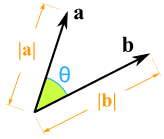Definition of

# Dot ProductA way of multiplying two vectors:

a · b = |a| × |b| × cos(θ)

Where || means "the magnitude (length) of"
And θ is the angle between the vectors

Example: the lengths of two vectors are 3 and 4, and the angle between them is 60°
So the dot product is:

a · b = 3 × 4 × cos(60°) = 3 × 4 × 0.5 = 6